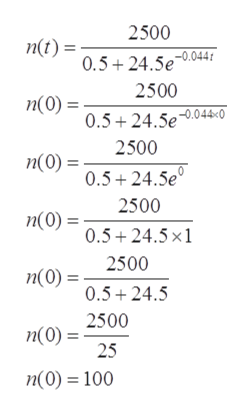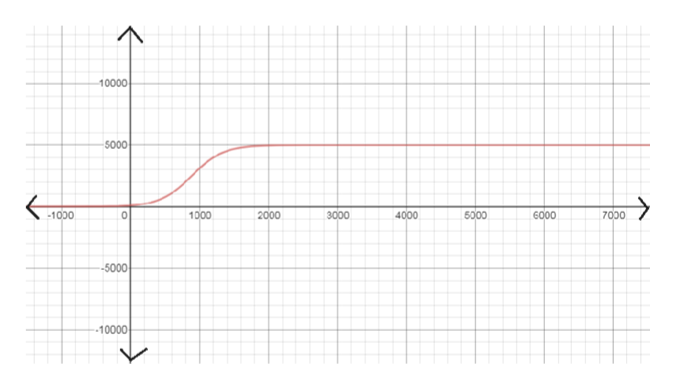The population of a certain species of bird is limited by the type of habitat required for nesting. The population behaves according to the logistic growth modeln(t) =2,5000.5 + 24.5e−0.044twhere t is measured in years.(a) Find the initial bird population.(b) Draw a graph of the function n(t).

Question

The population of a certain species of bird is limited by the type of habitat required for nesting. The population behaves according to the logistic growth model

n(t) =
 2,500 0.5 + 24.5e−0.044t

where t is measured in years.

(a) Find the initial bird population.
(b) Draw a graph of the function n(t).
Step 1

Given:

‘t’ is measured in the year.

Here, the equation of the logistic growth model:

Step 2

Part (a)

Finding the initial population of bird:

Here, t = 0help_outlineImage Transcriptionclose2500 nt)5+24.5e04f 2500 п(0) %3 0.5+24.5e.044«0° 2500 п(0) - 0.5+24.5e 2500 п(0) 0.5 24.5x1 2500 n(0)= 0.5 24.5 n(0)2500 25 n(0)=100 fullscreen
Step 3

Part (b)

The graph of the funct...help_outlineImage Transcriptionclose10000 5000 3000 5000 1000 2000 4000 6000 -1000 7000 -5000 10000 fullscreen

Want to see the full answer?

See Solution

Want to see this answer and more?

Our solutions are written by experts, many with advanced degrees, and available 24/7

See Solution
Tagged in

Calculus